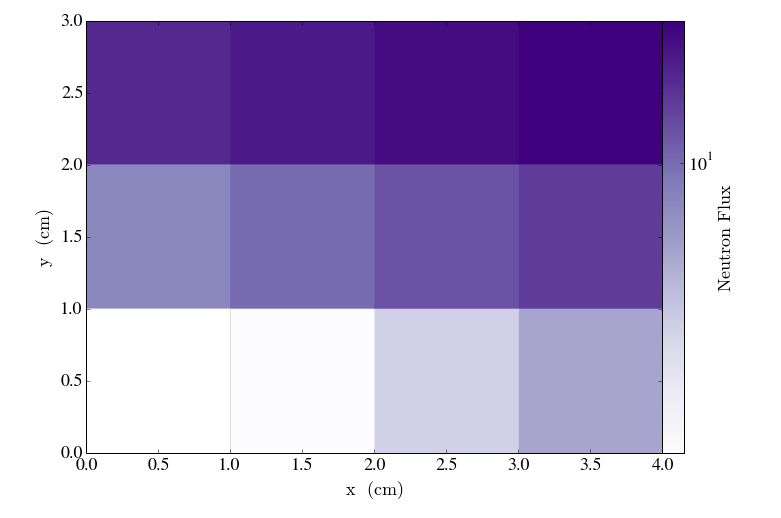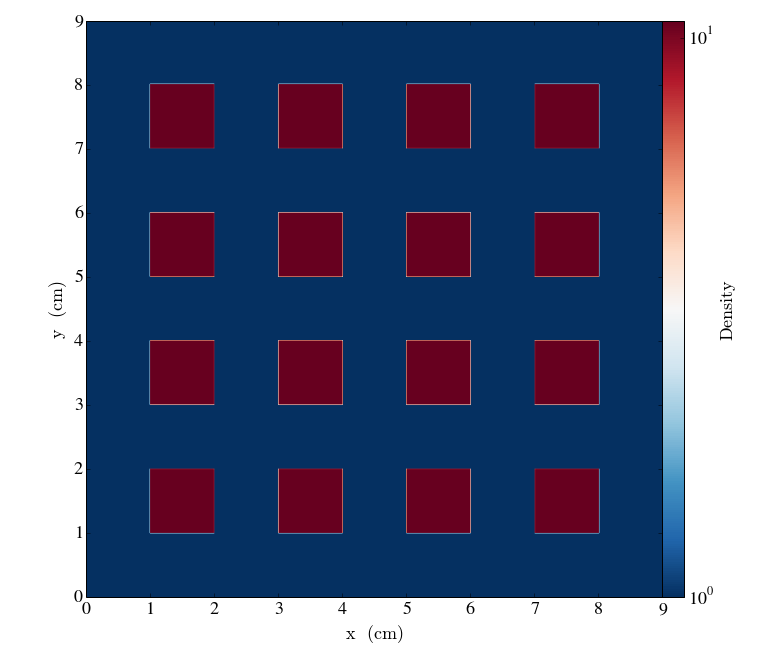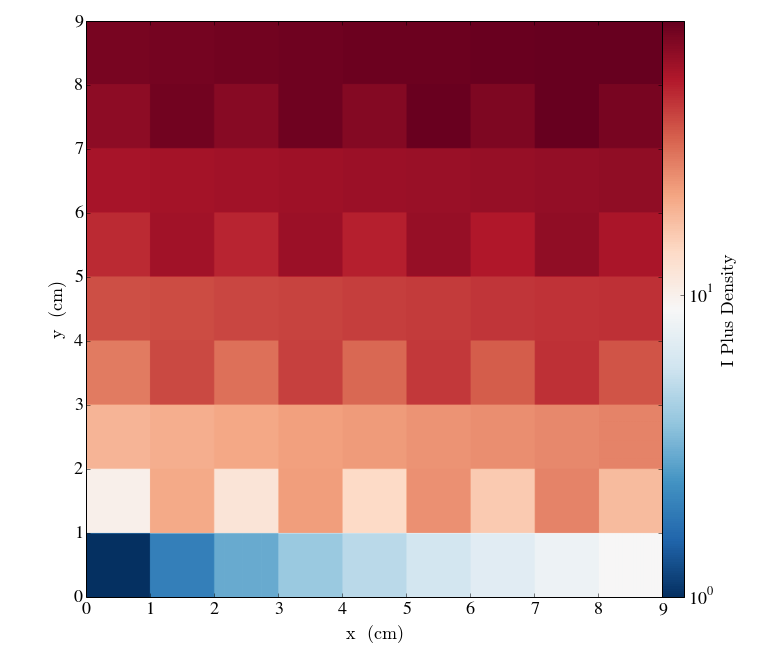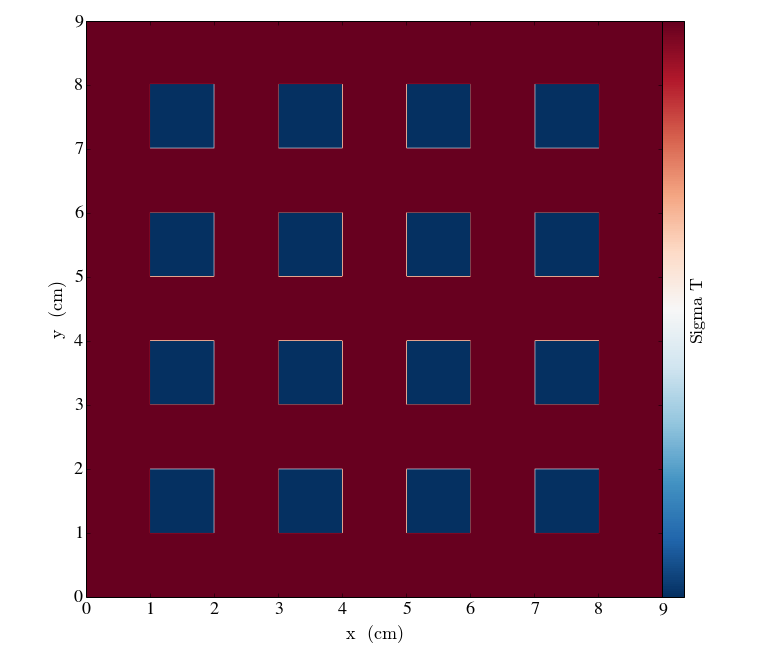# Mesh Basics¶

Download the full notebook.

07-mesh-basics

The PyNE mesh module provides generic mesh creation and manipulation capabilities. Both Cartesian and tetrahedral mesh are supported. PyNE meshes can be tagged with scalar and vector quantities and also PyNE Material objects. Tagged data can all be easily accessed with a common syntax.

Under the hood, the PyNE Mesh class is a wrapper around a MOAB mesh. A Mesh object stores a mesh instance, and additionally a PyNE MaterialLibrary object (to map PyNE Materials to mesh volume elements).

The notebook will provide some basic examples of PyNE mesh usage. First let's import the necessary components of PyNE and a few other things that will be explained in this notebook:

#### Known error: This notebook requires yt to visualize the results. Yt needs to be updated to work properly first. Updates are currently being made to yt's frontends to make this PyNE integration work.¶

In :
# Import some classes from pyne.mesh
from pyne.mesh import Mesh, NativeMeshTag, MetadataTag, ComputedTag
from pyne.material import Material, from_atom_frac
from pyne.xs.channels import sigma_t
import numpy as np

# import plotting utilities
from yt.config import ytcfg; ytcfg["yt","suppressStreamLogging"] = "True"
from yt.frontends.moab.api import PyneMoabHex8Dataset
from yt.mods import SlicePlot


# Basics¶

Let's make a simple Cartesian mesh that is 4 x 3 x 1:

In :
coords = [[0, 1, 2, 3, 4], [0, 1, 2, 3], [0,1]]
m = Mesh(structured=True, structured_coords=coords) # here "structured" means Cartesian


Tags -- sometimes known as fields -- are a way of storing data on a mesh. Both scalar and vector quantities can be tagged on each volume element of the mesh using PyNE NativeMeshTags. Here we create a scalar, floating point tag to describe the neutron flux on a mesh:

In :
m.neutron_flux = NativeMeshTag(size=1, dtype=float)


Some fictitious neutron flux data can now be applied to the mesh:

In :
m.neutron_flux[:] = [0.0, 2.0, 4.0, 6.0, 8.0, 10.0, 12.0, 14.0, 16.0, 18.0, 20.0, 22.0]


PyNE meshes are supported by the yt project. Here we use yt's plotting infrastructure to display the mesh tag that was just created:

In :
pf = PyneMoabHex8Dataset(m)
s = SlicePlot(pf, 'z', 'neutron_flux', origin='native')
s.set_cmap('neutron_flux', 'Purples')
s.display()The volume elements within the mesh are kept track of using the volume element index (i). The volume element index is defined on the range from 0 (inclusive) to the number of volumes in the mesh (exclusive).

The flux for the volume element i=7 can be retrieved (and similarly set) using the following syntax:

In :
m.neutron_flux

Out:
14.0

"Fancy" indexing can be used. This means that multiple indicies can be provided, yielding the tag value for each:

In :
m.neutron_flux[2, 3, 4]

Out:
array([ 4.,  6.,  8.])

Masking can be used to return only values that match some criteria. Here we request all flux values above 10.0:

In :
m.neutron_flux[m.neutron_flux[:] >= 10.0]

Out:
array([ 10.,  12.,  14.,  16.,  18.,  20.,  22.])

# PyNE Mesh + PyNE Materials¶

PyNE meshes can natively store PyNE Material objects. This can be used to represent discretized versions of geometries. First we create a new mesh, m2, that is a slightly larger mesh than our previous example. This one is 9x9x1:

In :
coords2 = [np.linspace(0,9,10), np.linspace(0,9,10), [0, 10]]
m2 = Mesh(structured=True, structured_coords=coords2)


Create a few materials that will be assigned to the mesh:

In :
fuel = from_atom_frac({'U235': 0.045, 'U238': 0.955, 'O16': 2.0}, density=10.7)
mod = from_atom_frac({'H1': 2.0, 'O16': 1.0}, density=1.0)


Loop through the mesh and assign materials in a reactor-like pattern:

In :
for i in range(len(m2)):
if (i+1)%2 != 0 and np.floor((i)/9)%2 != 0:
m2.mats[i] = fuel
else:
m2.mats[i] = mod


Plot the density. Here the fuel appears red (high density) and the moderator appears blue (low density).

In :
pf2 = PyneMoabHex8Dataset(m2)
s2 = SlicePlot(pf2, 'z', 'density', origin='native')
s2.set_cmap('density', 'RdBu_r')
s2.display()The materials that we've added to the mesh can be accesssed via the same syntax used for NativeMeshTags. The material on the the mesh volume element with index i=12 is retrieved:

In :
m2.mats

Out:
pyne.material.Material({80160000: 0.11852241570586383, 922350000: 0.03918773177732002, 922380000: 0.8422898525168162}, 269.905309039, 10.7, 3.0, {})

The attributes of this material can be accessed:

In :
m2.mats.density

Out:
10.7

Equivalently the density can be accessed via the generic tag syntax:

In :
m2.density

Out:
1.0

The same is true of the composition of the material (another attribute of the Material class):

In :
m2.comp

Out:
{10010000: 0.11191487328808077, 80160000: 0.8880851267119192}

In fact, all Material attributes are treated as tags. A list of all tags, including these material properties treated as tags is shown here:

In :
m2.tags.keys()

Out:
['sub_act',
'expand_elements',
'number_density',
'sub_tru',
'idx',
'density',
'comp',
'atoms_per_molecule',
'to_atom_frac',
'sub_lan',
'molecular_mass',
'mass',
'sub_fp',
'mult_by_mass',
'sub_ma',
'mass_density',
'metadata']

Computed tags (also known as "derived fields") are a way of creating a "virtual" tag. That is, computed tags to not require tag data to be actually stored on the mesh, or even computed ahead of time. When data is requested from a computed tag, it is calculated and returned on-the-fly (this is known as "lazy evaluation"). This convenient feature when dealing with extremely large data.

A computed tag is defined with a function, lambda (a.k.a an "anonymous" function), or any other callable object. The function must have the following interface:

def f(mesh, i):
"""mesh is a pyne.mesh.Mesh() object and i is the volume element index
to compute.
"""
# ... do some work ...
return anything_you_want


Computed tags are probably best-understood through example. Here is a simple, somewhat silly example that creates a computed tag i_plus_density which is simply the sum of the mesh volume element index i and density:

In :
m2.i_plus_density = ComputedTag(lambda mesh, i: i + mesh.density[i])


Plotting i_plus_density:

In :
pf3 = PyneMoabHex8Dataset(m2)
s3 = SlicePlot(pf3, 'z', 'i_plus_density', origin='native')
s3.set_cmap('i_plus_density', 'RdBu_r')
s3.display()Here is a more serious example which uses PyNE's cross section tools to compute the one-group total cross section $\sigma_t$ everywhere on the mesh.

In :
m2.sigma_t = ComputedTag(lambda mesh, i: sigma_t(mesh.mats[i], group_struct=[10.0, 1e-6], phi_g=[1.0]))

In :
pf4 = PyneMoabHex8Dataset(m2)
s4 = SlicePlot(pf4, 'z', 'sigma_t', origin='native')
s4.set_cmap('sigma_t', 'RdBu_r')
s4.display()This plot shows that the one-group total cross section is highest in the moderater -- all from a computed tag generated in one line of code!